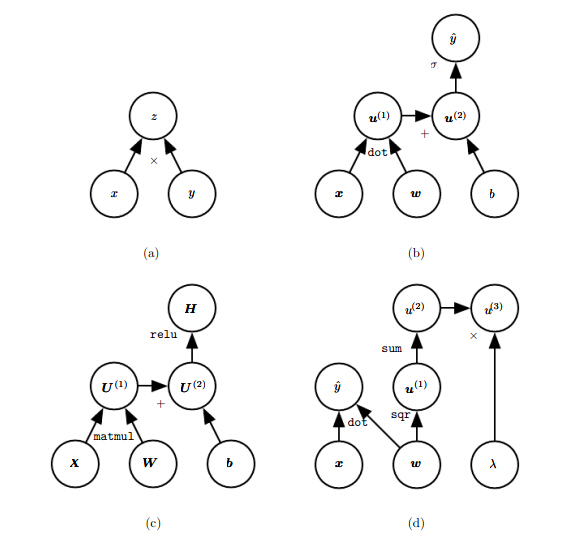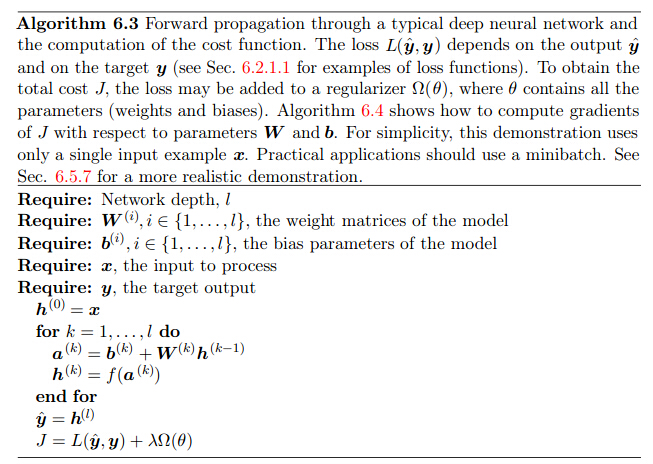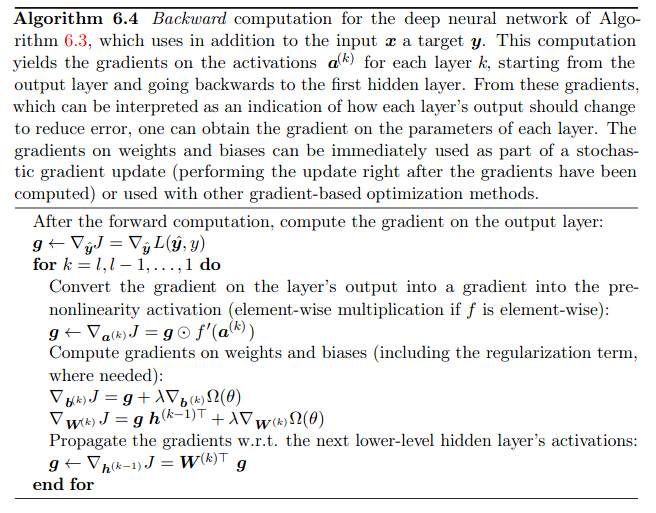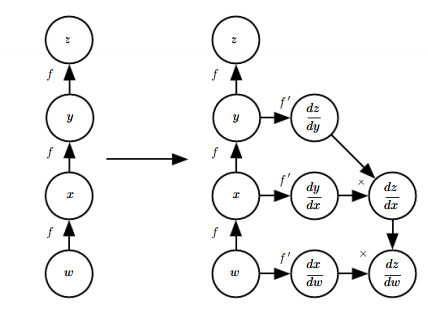# FNN基本概念

FNN理论上能够拟合各种不同类型函数表达。

FNN采取的思路是对输入x进行各种非线性变换，直到达到较好的效果。

# 基于梯度学习算法

## 价值函数

J(θ)=Ex,ypdatalogpmodel(y|x)

## 输出单元

FNN包括三种比较重要的层次，输入层常常表示为一维向量或者多维向量；中间层也叫隐藏层；输出层。

1. 线性单元，即输出表示为y^=WTh+b$\hat y=W^Th+b$，常用于回归
2. Sigmoid单元：即y^=σ(WTh+b)$\hat y=\sigma(W^Th+b)$，常用于二元分类
3. SoftMax单元：即z=Wh+b,softmax(zi)=exp(zi)jexp(zj)$z=W^h+b, softmax(z_i)=\frac{exp(z_i)}{\sum_j exp(z_j)}$，常用于多分类问题
4. 其他输出单元

# 隐藏单元

## 修正线性单元（ReLU）

1. ReLU的一个最大优势在于计算效率非常高。
2. 工程实际中常常将b设置为一个较小的整数，例如0.1
3. ReLU的问题在于如果某个实例的激活值为0，则该参数无法通过梯度的方法进行学习，因此有很多变形解决该问题
4. ReLU的泛化变形可以为h=g(z,α)=max(0,zi)+αmin(0,zi)$h=g(z,\alpha)=max(0, z_i) + \alpha min(0,z_i)， 即但激活值为负时，添加负数项$
5. 绝对值修正（Absolute Value Rectification）将参数α=1$\alpha=-1$
6. Leaky ReLU将参数设置为0.01
7. Pamametric ReLU 将α$\alpha$当做参数进行学习。
8. Maxout Units是更进一步的泛化，将z分成若干个组分别进行最大值操作，g(zi)=maxjG(i)zj$g(z_i)=max_{j \in G(i)} z_j$

## Sigmoid 单元

Sigmoid单元 g(z)=σ(z)$g(z)=\sigma(z)$

# 网络架构设计

FNN常见的网络架构设计是线性架构，即每一层的输入都是来自于其父节点，例如

h1=g1(Wx+b)h2=g2(W2h1+b)

1. 这类结构仅仅需要设计的参数包层数以及每一层的宽度，即隐藏节点的个数
2. 根据”统一近似理论“只要要拟合的函数满足一定测度分布，FNN就能表达出来。即FNN能拟合所有的映射，从有限维度到另外一个有效维度。
3. “天下没有免费的午餐”，虽然FNN能拟合所有的函数，但不能保证学习算法能够学习到相关参数。
4. FNN这种基础的线性结构，后续还会针对特定问题构造不同的网络结构，例如CNN、RNN等

# 反向传播算法

Back-Propagation反向传播算法是训练FNN最重要的方法，由于映射函数的复杂性，通过梯度学习算法进行求解时，梯度不好计算，因此研究学者提出通过BP算法进行梯度的传播求解。

1）通过前向传播计算网络中各个节点的激活值。
2）将梯度通过反向传播算法进行传播，得到各个参数的梯度值。
3）采用梯度下降法进行模型参数的更新。
4）迭代执行上述步骤，直到收敛。

## 可计算图1. 图a 表达的是 z=xy$z=x*y$
2. 图b 表达的是 y=σ(wx+b)$y=\sigma(w^x+b)$
3. 图c表达的是h=g(wx+b)$h=g(w^x+b)$
4. 图d表达的是y=wx+λ||w||$y=w^x+\lambda ||w||$

## 微分的链式法则

y=g(x)z=f(g(x))=f(y)dzdx=dzdydydx

xRm,yRny=g(x),gRmRnz=f(y),fRNRzxi=jzyjyjxi

## 反向传播算法应用于FNN## 符号表示

”符号-数值导数推导“：输入包括一个计算图和输入变量值，返回各个节点的梯度值，代表软件Torch和Caffe
“符号-符号导数推导”：输入包括一个计算图，通过增加额外的节点提供另外的符号表示进行梯度的推导，主要不同在于输出还是一个可计算图，用于计算各个节点的梯度。示例如下：# 总结

FNN是深度学习中最基础的网络结构，需要着重了解
1. FNN的基础网络结构，标准线性结构
2. 常用隐藏单元和输出单元
3. 模型训练算法-经典的BP算法
4. 了解常见开源实现BP算法的思路
5. 设计自己的网络结构并应用。

©️2019 CSDN 皮肤主题: 大白 设计师: CSDN官方博客